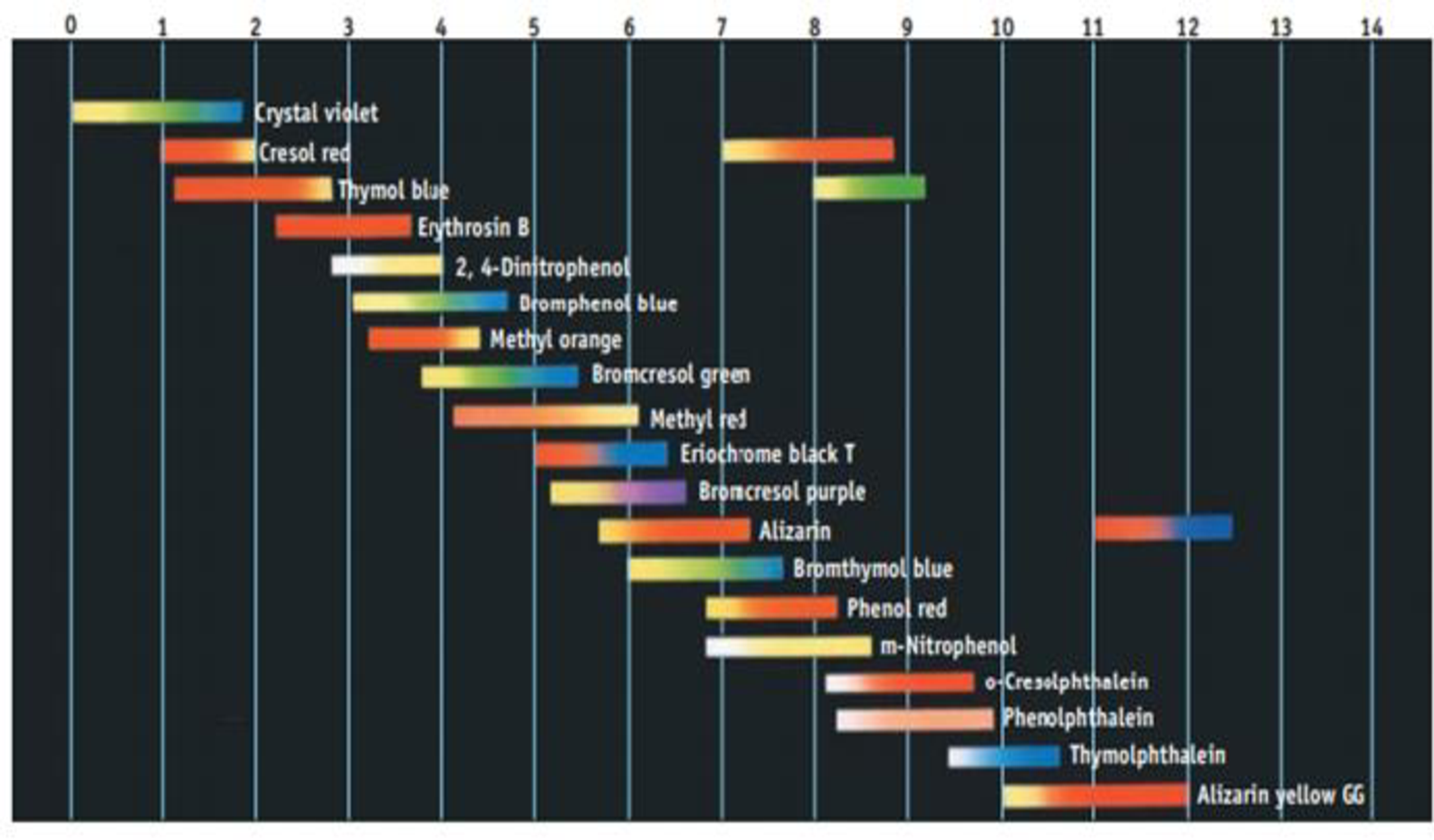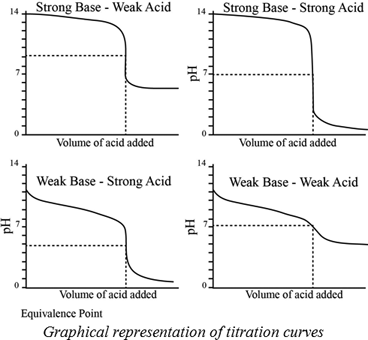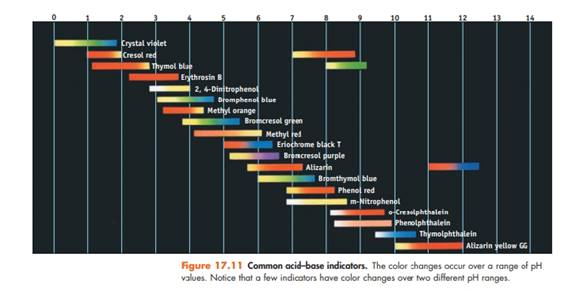# Using Figure 17.11, suggest an indicator to use in each of the following titrations: (a) The weak base pyridine is titrated with HCl. (b) Formic acid is titrated with NaOH. (c) Ethylenediamine, a weak diprotic base, is titrated with HCl. Figure 17.11 Common acid-base indicators. The color changes occur over a range of pH values. Notice that o few indicators hove color changes over two different pH ranges.### Chemistry & Chemical Reactivity

9th Edition
John C. Kotz + 3 others
Publisher: Cengage Learning
ISBN: 9781133949640

#### Solutions

Chapter
Section### Chemistry & Chemical Reactivity

9th Edition
John C. Kotz + 3 others
Publisher: Cengage Learning
ISBN: 9781133949640
Chapter 17, Problem 35PS
Textbook Problem
47 views

## Using Figure 17.11, suggest an indicator to use in each of the following titrations: (a) The weak base pyridine is titrated with HCl. (b) Formic acid is titrated with NaOH. (c) Ethylenediamine, a weak diprotic base, is titrated with HCl.Figure 17.11 Common acid-base indicators. The color changes occur over a range of pH values. Notice that o few indicators hove color changes over two different pH ranges.

a)

Interpretation Introduction

Interpretation:

The weak base pyridine is titrated with HCl, an indicator used has to be determined.

Concept introduction:

A titration is one of the most useful ways of determining accurately the quantity of an acid, a base or some other substances in a mixture.

The pH at the equivalence point of a strong acid –strong base titration is 7.

A weak acid titrated with a strong base leads to a pH > 7 at the equivalence point.

A weak base titrated with a strong acid leads to pH < 7 at the equivalence point.Indicators:

A chemical substances which gives a visible change  in the titration.

Some important indicators are as follow.### Explanation of Solution

Pyridine is a weak base and has a pkb= 8.82. Titrating a weak base with a strong acid HCl.

Therefore, the resultant solution pH will be less than the 7

b)

Interpretation Introduction

Interpretation:

Formic acid is titrated with NaOH , an indicator used has to be determined.

Concept introduction:

A titration is one of the most useful ways of determining accurately the quantity of an acid, a base or some other substances in a mixture.

The pH at the equivalence point of a strong acid –strong base titration is 7.

A weak acid titrated with a strong base leads to a pH > 7 at the equivalence point.

A weak base titrated with a strong acid leads to pH < 7 at the equivalence point.Indicators:

A chemical substances which gives a visible change  in the titration.

Some important indicators are as follow.c)

Interpretation Introduction

Interpretation:

Ethylene diamine, a weak diprotic base, is titrated with HCl, an indicator used has to be determined.

Concept introduction:

A titration is one of the most useful ways of determining accurately the quantity of an acid, a base or some other substances in a mixture.

The pH at the equivalence point of a strong acid –strong base titration is 7.

A weak acid titrated with a strong base leads to a pH > 7 at the equivalence point.

A weak base titrated with a strong acid leads to pH < 7 at the equivalence point.Indicators:

A chemical substances which gives a visible change  in the titration.

Some important indicators are as follow.### Still sussing out bartleby?

Check out a sample textbook solution.

See a sample solution

#### The Solution to Your Study Problems

Bartleby provides explanations to thousands of textbook problems written by our experts, many with advanced degrees!

Get Started

Find more solutions based on key concepts
In naming ionic compounds, we always name the second.

Introductory Chemistry: A Foundation

What are the base-pairing rules for DNA? a. AG, TC b. AT, GC c. AC, TG d. AA, GG, CC, TT

Biology: The Unity and Diversity of Life (MindTap Course List)

Which is true of very low-fat diets? a. They may lack essential fatty acids and certain vitamins. b. They may b...

Nutrition: Concepts and Controversies - Standalone book (MindTap Course List)

In the following diagram, designate each daughter cell as diploid (2n) or haploid (n).

Human Heredity: Principles and Issues (MindTap Course List)

A uniform magnetic field is directed along the x axis. For what orientation of a flat, rectangular coil is the ...

Physics for Scientists and Engineers, Technology Update (No access codes included)

How is a forced wave different from a free wave?

Oceanography: An Invitation To Marine Science, Loose-leaf Versin